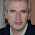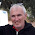## 19 jul. 2012

### Hierarchical Cluster Analysis (ChemoSpec) - 02

This is the second derivative spectra of the raw spectra we have sawn in the post: "Hierarchical Cluster Analysis (ChemoSpec) - 01". In that post we saw some clusters, but the distance between the clusters was not high, so it was clear that some math treatment should be applied to remove baseline shifts and to increase the differences between the clusters as much as possible.
Well, let’s see now the HCA in this case:

Well, now it looks much better, Olive samples in one cluster, and sunflower oil samples in another. We can see also two sub-cluster in the sunflower samples. Looking to the spectra we can see some reasons for that more clearly now. That will be treated in the next post.

#### 9 comentarios:

1.Could you please explain the math treatment that was done since the results are much much better

excellent work

2.I convert the raw spectra to second derivative using a Chemometric software called Win Isi, but you can use others like Unscrambler,...., after that these softwares can export the spectrum to a TXT file son I can import it into R.
These softwares calculate the derivative based in the segment-Gap concept. Gap is always cero and I used a segment of 10. You can go to the labes "derivadas", where I explain this concept, in spanish (I,ll translate these post in a near future), but there are some drawings which can help. Of course could be possible to develop a function in R to do the same.
Probably you will get the same results using the SG filters in R where you can configure them for first,second derivative, third.....

3.In R, you can use the function sgolayfilt() in package signal to get the derivatives. If you are using ChemoSpec, the spectral data is in SpectraObject\$data, so you can make a copy of SpectraObject and then replace the \$data with the derivatives, then work from there. For the first derivative you would use something like SpectraObject\$data <- sgolayfilt(SpectraObject\$data, m = 1) but as \$data is a matrix, you would have to use a plyr or apply method to "loop" over each row of the matrix.

Thanks JR!

1.Thanks to you Bryan for reading these posts and give to us good advices to know better your package and R in general. I will practice with the points you say.

2.I'm glad you made the post about using derivatives with HCA, as I had not really thought about that much but I think I have some data sets where I can use that idea.

3.The derivative in the sgolayfilt() function does not take into account the increments in the x (wavelength in our case).
Based on
http://r.789695.n4.nabble.com/how-to-calculate-derivative-td3054546.html
a simple R function for the first derivative would be
"d1" <-
function(x,y)
{
deriv <- diff(y)/diff(x)
times <- (x[-1] + x[-length(x)])/2
cbind(x=times,y=deriv)
}

Agus

4.Thank you very much for these answers, It is really intersting

5.José Ramón,
I'm interested on this approach as it looks like some work done for classifying time series of ndvi. The
improvement at using the derivative (BTW, why the 2ond and not the 1st? was there an improvement when
using the 1st?) is very interesting. Are you aware of articles using this approach in your field?
Thanks
Agus

1.Hi,
In some cases I prefer much more the second derivative than the first one. First to understand the spectra and the bands. We usually are used to see the peaks and try to understand wich molecules are absorbing to give that peaks. With the first derivative the positive peaks in the raw spectrum become a zero crossing and the spectrum becomes dificult to understand.
With the second one , the positive peak in the row spectrum becomes a negative peak, and new bands (also negative) can appear , before overlapped by the bigger and broad bands. The resolution of the peaks also increase.
You have to ignore some shoulders that appear on the sides of these peaks.
The problem can be that if the quality of the raw spectra is poor(high absorbances, noise,...), the second derivative increase the noise.
Second derivative is very useful for discriminant analysis (for example in the pharmaceutical industry to identify and qualify excipients and active ingredients), and is very usefull with liquids (working in transmitance). With solids, due to the scatter, sometimes can be better the first derivative together with another anti-scatter correction like SNV , MSC,.... I use this aproach more for quantification.
Anyway if the particle size is small second derivative can work very well even for quantitative analysis.